Search IntMath
Close

450+ Math Lessons written by Math Professors and Teachers

5 Million+ Students Helped Each Year

1200+ Articles Written by Math Educators and Enthusiasts

Simplifying and Teaching Math for Over 23 Years

# Is the Gateway Arch a Parabola?

By Murray Bourne, 06 May 2010

The Gateway Arch in St Loius, Mo., USA, is part of the Jefferson National Expansion Memorial.

The shape of the arch certainly looks like a parabola, but is it?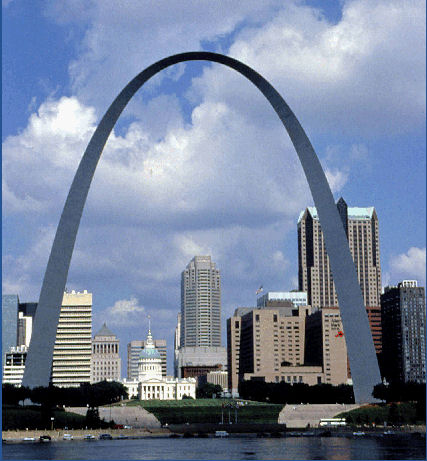The Gateway Arch, St Loius, USA [Image source]

We'll model the arch using a parabola and see how well it fits.

I drew a set of x-y axes and a regular grid on top of the photograph.

Next, I determined the values of y for x ranging from -3 to 3 (using a much finer grid than the one shown). We can see the resulting points (in magenta color) in the next image. Note the points are along the (approximate) center line of the arch (not the inner or outer edge).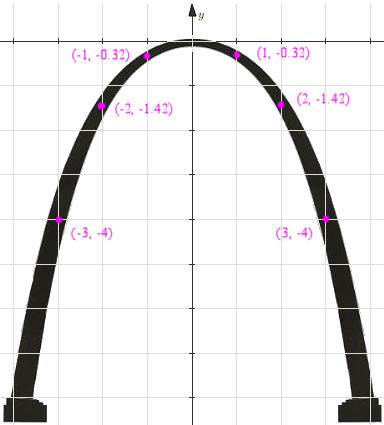So my aim is to find the parabola that passes through the Origin (0, 0) and the points (-3, -4) and (3, -4). (I choose these for convenience).

I know the equation for my parabola will have a negative in front, since it is inverted (upside-down).

I can find the equation for this parabola in several different ways. One is to recall the general form for a parabola:

y = ax2 + bx + c

We have 3 points and 3 unknowns, so we just substitute them in:

Substituting (0,0) gives:

0 = 0 + 0 + c

So c = 0.

Next, substitute (−3, −4) and (3, −4) to give 2 equations in 2 unknowns:

−4 = 9a − 3b (1)

−4 = 9a + 3b (2)

Adding (1) and (2) gives:

−8 = 18a

So a = −4/9

Substituting this back into either (1) or (2) gives:

b = 0

So the parabola we are looking for is: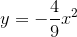Here is the graph of that parabola.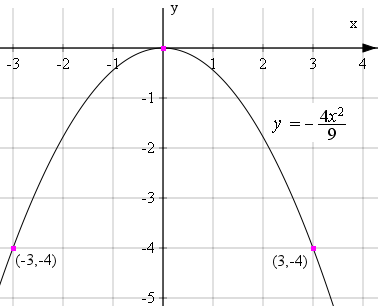It looks quite good. It passes through the Origin and the points (-3, -4) and (3, -4). But how does it match up with the Gateway Arch?

Here is the parabola next to the points we found above (when x = -3, -2, -1, 0, 1, 2, 3):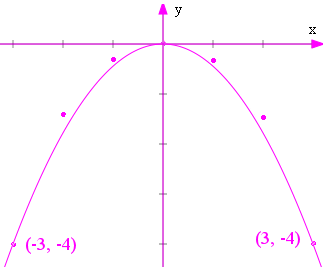Our parabola has missed going through the other points (the ones where x = −2, −1, 1 and 2)!

Comparing our parabola to the photo, we can see (especially near the waterline at the bottom) the shape of the arch is not really a parabola at all.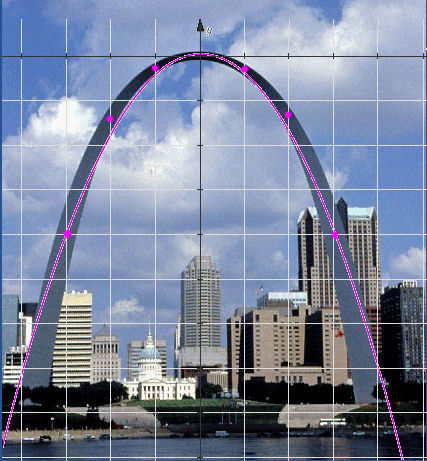## Another Curve Needed

The Finnish-American designer of the Gateway Arch, Eero Saarinen, knew that a parabola was not the best shape for such an arch.

Arches have been used throughout history for bridge and roof supports, since they are good at directing forces downwards, rather than outwards, thus reducing the chance of collapse.

An arch shape that is often used is the catenary.

A catenary is the shape made by a chain that is freely hanging between 2 supports.Chain hanging on a log. [Image source]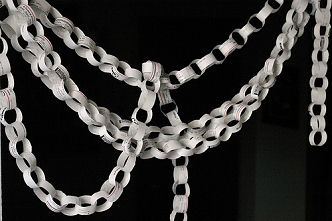Paper chains [Image source]

## Catenaries

A caternary is an interesting curve. It is the average of the y-values of an exponentially decreasing curve and an exponentially increasing curve.

A simple example is: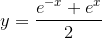The first part of the numerator, e−x, decreases exponentially as x increases, while the second part, ex, increases exponentially as x increases. (See more on exponential curves.).

Here are the two curves on the same set of axes.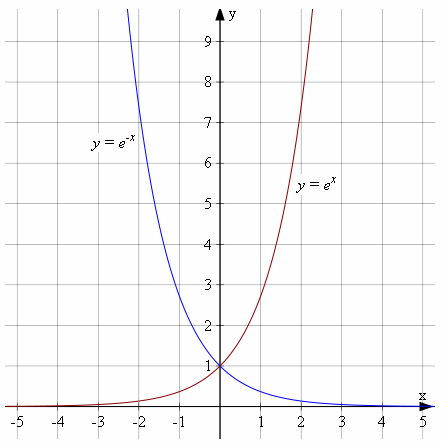We add the y-values of the 2 exponential curves together and divide by 2 (in other words, we find the average of the y-values) to give us our simple catenary (in black) .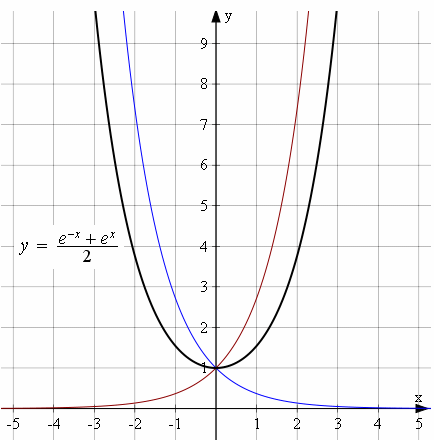Let's now compare the shape of a catenary (in black) to the shape of a parabola (in magenta).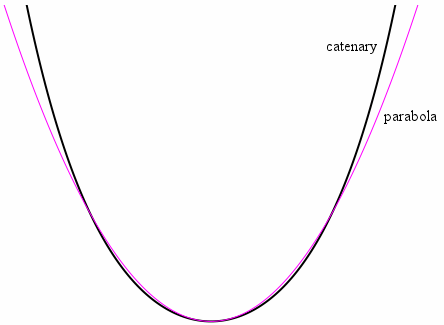We see they are not quite the same, and perhaps we are getting somewhere.

## [Aside] Cosh

This is not important for this discussion but there is another way of writing the equation we've just been discussing.This is an example of a hyperbolic function and you may meet the notation cosh later. It is called "cosh" because in some ways, it behaves similarly to the "cosine" function from trigonometry. The "h" in "cosh" comes from "hyperbolic".

We can write: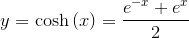See more on hyperbolic functions.

Back to the topic.

## Flattened Catenaries

Now, a catenary is the shape we see when there is a chain of constant thickness hanging between 2 fixed points. (The word "catenary" comes from the same word as "chain".)

But if the chain is thinner in the middle, it has a slightly different shape (it is flatter). Since the Gateway Arch is thinner at the top than it is near the base, the architect chose a flattened catenary, whose equation has the form: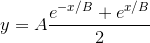Now, in our example there will be a negative in front of our equation (since the catenary is inverted) and we also will need to add A to our equation so the curve passes through (0,0) (otherwise it will pass through (0, -A)).

So we are looking for an equation of the following form, that passes through (−3, −4) and (−2, −1.42):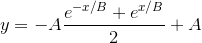Using a computer algebra system, we solve the above system of equations and obtain the following equation.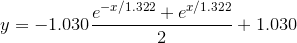Here is the plot of that curve superimposed on the Gateway Arch (in light green). The parabola we found earlier (in magenta) is there for comparison. We can see our catenary follows the shape of the bridge quite closely, especially at the bottom.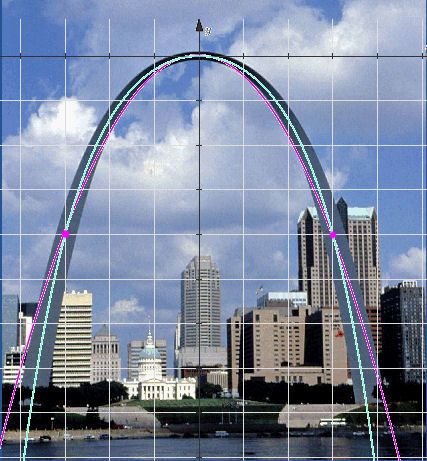## Conclusion

This article has shown the Gateway Arch is not a parabola. Rather, it is in the shape of a flattened (or weighted) catenary, which is the shape we see if we hang a chain that is thin in the middle between two fixed points.

We have also seen how to go about modeling curves to find the equation representing such curves.See the 48 Comments below.

### 48 Comments on “Is the Gateway Arch a Parabola?”

1. Jessy George says:

This is really intersting am going to share with my students ,as they can adapt this method to check

keep up your good work

Thank you Murray

2. Farzin says:

Very interesting. I always thought it was a parabolla.

Thanks

3. Rina King says:

Thanks a ton for this Rika! It is a great example to show learners why in science we need to show a directly proportional relationship,ie resolve it into a straight ine - any other relationship cannot be assumed.

4. Anah says:

We just finished a project in calculus II. The subject was to find the best model for the cumulative new cases of AIDS and the epidemic curve in the US. I wonder if there is an ideal model taking into account different factors such as geographic, bio...Ifsomeone has an idea, I will be interested.
Thanks for your work

5. Murray says:

Hi Anah. This earlier article may help you: H1N1 and the Logistic Equation.

6. payal says:

Thanks for the information.I have learned lot many new things from this article.I would be happy if you send me more such information.
Thanks again

7. rishabh says:

really very interesting!!!!!!!!!!!!!!!!!

8. abramovich says:

interesting indeed

9. harold2 says:

I really appreciated it!
Congratulations for this excellent post!

10. Leonor Adio Batan says:

Thank you. I have learned a lot. I thought it was a parabola...nice..

11. Nikki says:

Thank you. I was recently reading a book that described my beloved Arch as a "Half-parabola." That can't be right, I thought, I don't believe the Arch is a parabola at all, and it certainly isn't a half of one. You have confirmed my suspicions. Thank you.

12. ?mega£ither says:

D: great, now I have to tell my awesome 7th grade algebra honors teacher she's wrong (advanced class)

great explanation . . . i am expecting much of such these facts instead of boring old concepts. add more and more applications with as much less theory as possible... thank you.

14. Nick says:

Shouldn't you be comparing various curve types against an architectural drawing of the arch, as your photograph will have perspective distortion.

15. Murray says:

@Nick: You're right - there will be some parallax error for the photograph.

Here is the original architectural drawing of the Gateway Arch: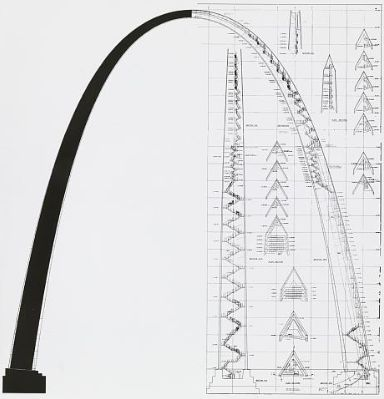[Source: Library of Congress]

Here's a catenary placed over that drawing: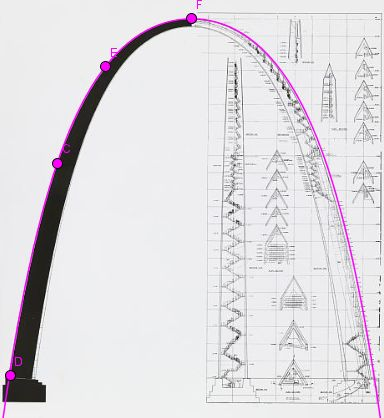I drew the catenary using GeoGebra. It turned out to be: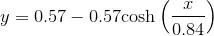For comparison, here is a parabola through the extremities of the arch.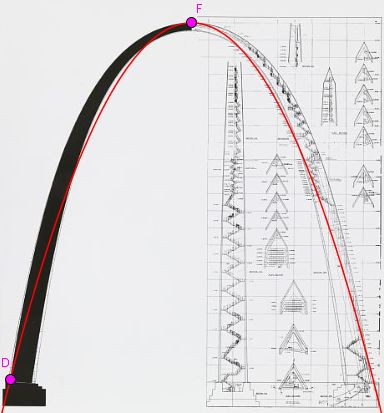So while there may have been some parallax issues in my earlier analysis, I believe it was insignificant, especially since the photo I chose had the arch directly facing the camera, and after all, a "squashed" catenary is still (almost) a catenary, and a "squashed" parabola is (almost) still a parabola, as long as the distortion is along the axis of the curve.

Edit (Nov 2019): The center line of the arch is a catenary (not the outer edge as per my diagram above). But the point still holds - parallax error is not significant in the chosen photo.

16. Francesca says:

What program did you use to place the plane of coordinates in front of the picture?

17. Murray says:

@Francesca: I'm using GeoGebra (and/or Scientific Notebook) to create the coordinate plane image, then I place a transparent screen shot from there over the image using PaintShop Pro, which is similar to Photoshop).

18. Francesca says:

Thanks a lot!

19. Francesca says:

What is the computer algebra system that you used to solve the simultaneous equations for the hyperbolic case?

20. Murray says:

@Francesca: I used Scientific Notebook to solve that system. You could probably use Wolfram|Alpha to get the solution as well.

BTW, it was a "hyperbolic cosine" (or "catenary") case, which is different from a "hyperbola".

21. Francesca says:

What further calculations can you do once you have the function for the arch?

22. Murray says:

@Francesca: Probably the main reason for finding such a function would be to find height of the arch at various points along the ground underneath it.

One practical example could be if you needed to erect scaffolding under one section (for maintenance, or whatever), then how high would it need to be at various points.

23. Husna says:

Hello Murray:) it's an interesting sharing. Thank you very much. May I request for your email address as there's some question that I would love to ask in detail.

24. Murray says:

@Husna: If your question is about parabolas or catenaries, it's probably best to ask here, then everyone can benefit.

If not, perhaps one of the categories in the IntMath Forum would be appropriate for your question. Once again, other readers would benefit from the discussion.

25. Me says:

@Murray:I tried to proof the shape of the arch by using the same way as yours, using my own picture. Huhu But I failed to draw the parabolic shape. The size of the parabola is smaller than the arch, I mean very small..may I know, why is this happening

26. Murray says:

Hello again, Husna. What are you using to draw the parabola? What image editing software are you using?

27. Me says:

@murray Oh so I have to use image editing software?sorry didnt know about it😓😓😓im just using geogebra..so sorry

28. Star says:

Hey murray.how could we derive to the flattened catenary formula manually without using software?tq

29. Murray says:

@Star: I don't think there's a "nice" algebraic way to solve it. I believe you would need to do it numerically.

30. Star says:

@Murray: Would you mind to show it how

31. Murray says:

Well, the 2 equations in 2 unknowns from the known values (-3, -4) and (-2, -1.42) are: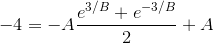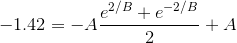Multiplying both through by 2 gives: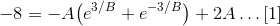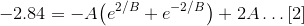Subtract  from :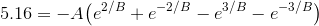You still have 2 unknowns, but could assume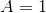(for now) and solve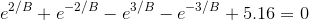Using just a few steps of Newton's Method gives you close to the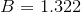I had before.

Then you would need to do some juggling (trial and error?) with A.

Much easier and more logical to get a computer to do it.

32. Star says:

tq for showing..just wondering why you choose values (-3,-4) and (-2,-1.42)..could it be other point as well?

33. April says:

Im interested in finding height of the arch at various points along the ground underneath the arch as u I mentioned. But im not sure how can I do it

34. Murray says:

@April: You just need to substitute in values of x) which are distances along the ground) to get the height h at that point.

For example, at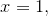we'll be atThis means we are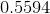units below the horizontal passing through the top of the arch. Assuming the arch is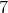units, high, the height there is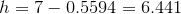35. Murray says:

@Star: Yes, I could have chosen any other points around the catenary. They just seemed convenient at the time.

36. Jan says:

Great article.

See also construction Sagrada familia in Barcelona. I assume construction based on same principals.
Constructions without side forces are intrisic strong.

37. The STEM Around Us | Casio Education Blog says:

[…] and trying to mathematically represent - is it a parabola? (In fact, it is NOT a parabola, but rather a flattened catenary). (Cool 3D mathematical model […]

38. Parabola’s in real life – Algebra 2017 says:
39. Flyover Comedy Festival: Angela Smith - St. Louis Independent Comedy says:

[…] as it is wide, you know! Did you know that? That the Arch is as TALL as it is WIDE?? It’s a parabola.”  The Arch should be paying me for this […]

40. Eric says:

Please Guys i will like to ask help on parabola dish Measurement i want a 40 feet dish Guys can you help me with the measurement ? i want to know the Depth of 40 feet dish

Diameter = 40 feet
Depth =
Focal length =

41. Murray says:

@Eric: This would be a good question for the IntMath Forum. Here's the appropriate section: Plane Analytic Geometry forum.

42. Lili says:

Hi! I wanted to know that if the Gateway Arch was a parabola, what would the equation be? For me, the roots came to be (4,0) and (-4,0). The y-intercept was (0,5). My vertex form didn't really work, but would standard form, or factored form work better?
Thank you!!

43. Murray says:

@Lili: Please see the early part of the article where I show how to find the equation of the parabola.

44. Danish says:

Hi murray,

on comment 31, you say
"Well, the 2 equations in 2 unknowns from the known values (-3, -4) and (-2, -1.42) are:"

My question is , how did you get the point (-2, -1.42)?

45. Murray says:

@Danish - The points chosen for the analysis came from overlaying a grid over the image of the arch, and reading it from the grid. The grid I used had a lot more precision than the one I used for the image in the article!

46. The Best Activities for Analyzing Quadratic Equations – ChalkDoc | Math Activities and Assessments says:

[…] P.S. Yes, the header image is of the Gateway Arch in St. Louis.  No, it’s not really a parabola.  It’s admittedly more complicated than that… […]

47. Gregory says:

The hyperbolic cosine curve (weighted, of course) represents the CENTROID curve of the Gateway arch, and hence, lies within the arch, not on the external surface of the arch. This can be read on the mathematical section of the Wikipedia article on the Gateway Arch. The outer curve and inner curve are demarcated by triangles normal (perpendicular) to the centroid curve. The equilateral edge length at the base of the arch is 54 feet and at the top of the arch is 17 feet. Between the two triangles the area of each triangle varies directly with height above the ground. The centroid curve lies 1/3 of the way from the outer base of the each triangle, and hence, 2/3 of the way to the inner point of the triangle.

48. Murray says:

@Gregory: Thank you so much for pointing this out. I have amended the post accordingly.

### Comment Preview

HTML: You can use simple tags like <b>, <a href="...">, etc.

To enter math, you can can either:

1. Use simple calculator-like input in the following format (surround your math in backticks, or qq on tablet or phone):
a^2 = sqrt(b^2 + c^2)
(See more on ASCIIMath syntax); or
2. Use simple LaTeX in the following format. Surround your math with $$ and $$.
$$\int g dx = \sqrt{\frac{a}{b}}$$
(This is standard simple LaTeX.)

NOTE: You can mix both types of math entry in your comment.